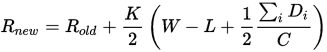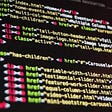# An extra weekRnew and Rold are the player’s new and old rating respectively, Di is the opponent’s rating minus the player’s rating, W is the number of wins, L is the number of losses, C = 200 and K = 32.

# Step 4: Testing the model and iterating

`import chessimport chess.pgnimport chess.uciboard = chess.Board()pgn = open("data/game1.pgn")game = chess.pgn.read_game(pgn)engine = chess.uci.popen_engine("stockfish")engine.uci()info_handler = chess.uci.InfoHandler()engine.info_handlers.append(info_handler)for move in game.main_line():engine.position(board) engine.go(movetime=2000) evaluation = info_handler.info["score"].cpif not board.turn:  evaluation *= -1  print move print evaluation board.push_uci(move.uci()) print board`

## Game 3 — I play White and checkmate Black in 26 moves

`import chessimport chess.pgnimport chess.uciboard = chess.Board()pgn = open("data/rickymerritt1_vs_maxdeutsch_2017-10-14_analysis.pgn")test_game = chess.pgn.read_game(pgn)engine = chess.uci.popen_engine("stockfish")engine.uci()info_handler = chess.uci.InfoHandler()engine.info_handlers.append(info_handler)prev_eval = 0diff = 0for move in test_game.main_line():print moveengine.position(board) engine.go(movetime=2000) evaluation = info_handler.info["score"].cpif board.turn:  if prev_eval - evaluation > 0.3:   print "bad move"  else:   print "good move"  if not board.turn:  evaluation *= -1  if evaluation - prev_eval > 0.3:   print "bad move"  else:   print "good move"   prev_eval = evaluation  board.push_uci(move.uci()) print board`
`def convertLetterToNumber(letter): if letter == 'K':  return '100000000000' if letter == 'Q':  return '010000000000' if letter == 'R':  return '001000000000' if letter == 'B':  return '000100000000' if letter == 'N':  return '000010000000' if letter == 'P':  return '000001000000' if letter == 'k':  return '000000100000' if letter == 'q':  return '000000010000' if letter == 'r':  return '000000001000' if letter == 'b':  return '000000000100' if letter == 'n':  return '000000000010' if letter == 'p':  return '000000000001' if letter == '1':  return '000000000000' if letter == '2':  return '000000000000000000000000' if letter == '3':  return '000000000000000000000000000000000000' if letter == '4':  return '000000000000000000000000000000000000000000000000' if letter == '5':  return '000000000000000000000000000000000000000000000000000000000000' if letter == '6':  return '000000000000000000000000000000000000000000000000000000000000000000000000' if letter == '7':  return '000000000000000000000000000000000000000000000000000000000000000000000000000000000000' if letter == '8':  return '000000000000000000000000000000000000000000000000000000000000000000000000000000000000000000000000' if letter == '/':  return ''def convertToBB(board): bitBoard = '' board = str(board.fen()).split(' ') for letter in board:  bitBoard = bitBoard + convertLetterToNumber(letter) return bitBoardprint convertToBB(board)`
`from __future__ import absolute_importfrom __future__ import divisionfrom __future__ import print_functionimport argparseimport sysfrom tensorflow.examples.tutorials.mnist import input_dataimport tensorflow as tfFLAGS = Nonedef main(_):  # Import data  mnist = input_data.read_data_sets(FLAGS.data_dir, one_hot=True)# Create the model  x = tf.placeholder(tf.float32, [None, 784])  W = tf.Variable(tf.zeros([784, 10]))  b = tf.Variable(tf.zeros())  y = tf.matmul(x, W) + b# Define loss and optimizer  y_ = tf.placeholder(tf.float32, [None, 10])# The raw formulation of cross-entropy,  cross_entropy = tf.reduce_mean(      tf.nn.softmax_cross_entropy_with_logits(labels=y_, logits=y))  train_step = tf.train.GradientDescentOptimizer(0.5).minimize(cross_entropy)sess = tf.InteractiveSession()  tf.global_variables_initializer().run()  # Train  for _ in range(1000):    batch_xs, batch_ys = mnist.train.next_batch(100)    sess.run(train_step, feed_dict={x: batch_xs, y_: batch_ys})# Test trained model  correct_prediction = tf.equal(tf.argmax(y, 1), tf.argmax(y_, 1))  accuracy = tf.reduce_mean(tf.cast(correct_prediction, tf.float32))  print(sess.run(accuracy, feed_dict={x: mnist.test.images,                                      y_: mnist.test.labels}))if __name__ == '__main__':  parser = argparse.ArgumentParser()  parser.add_argument('--data_dir', type=str, default='/tmp/tensorflow/mnist/input_data',                      help='Directory for storing input data')  FLAGS, unparsed = parser.parse_known_args()  tf.app.run(main=main, argv=[sys.argv] + unparsed)`
`from __future__ import absolute_importfrom __future__ import divisionfrom __future__ import print_functionimport argparseimport sysfrom tensorflow.examples.tutorials.mnist import input_dataimport tensorflow as tfFLAGS = Nonedef main(_):  # Import data  mnist = input_data.read_data_sets(FLAGS.data_dir, one_hot=True)# Create the model  x = tf.placeholder(tf.float32, [None, 784])  W = tf.Variable(tf.zeros([784, 10]))  b = tf.Variable(tf.zeros())  y = tf.matmul(x, W) + b# Define loss and optimizer  y_ = tf.placeholder(tf.float32, [None, 10])# The raw formulation of cross-entropy,  cross_entropy = tf.reduce_mean(      tf.nn.softmax_cross_entropy_with_logits(labels=y_, logits=y))  train_step = tf.train.GradientDescentOptimizer(0.5).minimize(cross_entropy)sess = tf.InteractiveSession()  tf.global_variables_initializer().run()  # Train  for _ in range(1000):    batch_xs, batch_ys = mnist.train.next_batch(100)    sess.run(train_step, feed_dict={x: batch_xs, y_: batch_ys})# Test trained model  correct_prediction = tf.equal(tf.argmax(y, 1), tf.argmax(y_, 1))  accuracy = tf.reduce_mean(tf.cast(correct_prediction, tf.float32))  print(sess.run(accuracy, feed_dict={x: mnist.test.images,                                      y_: mnist.test.labels}))if __name__ == '__main__':  parser = argparse.ArgumentParser()  parser.add_argument('--data_dir', type=str, default='/tmp/tensorflow/mnist/input_data',                      help='Directory for storing input data')  FLAGS, unparsed = parser.parse_known_args()  tf.app.run(main=main, argv=[sys.argv] + unparsed)`

# Part 1: Importing the necessary libraries and helper functions

`from __future__ import absolute_importfrom __future__ import divisionfrom __future__ import print_functionimport argparseimport sysfrom tensorflow.examples.tutorials.mnist import input_dataimport tensorflow as tf`

# Part 2: Reading the dataset

`FLAGS = Nonedef main(_):  # Import data  mnist = input_data.read_data_sets(FLAGS.data_dir, one_hot=True)`
`from __future__ import absolute_importfrom __future__ import divisionfrom __future__ import print_functionimport argparseimport sysfrom tensorflow.examples.tutorials.mnist import input_dataimport tensorflow as tfFLAGS = Nonedef main(_):  # Import data  mnist = input_data.read_data_sets(FLAGS.data_dir, one_hot=True)    print(mnist.test.images)  if __name__ == '__main__': parser = argparse.ArgumentParser() parser.add_argument('--data_dir', type=str, default='/tmp/tensorflow/mnist/input_data',       help='Directory for storing input data') FLAGS, unparsed = parser.parse_known_args() tf.app.run(main=main, argv=[sys.argv] + unparsed)`

# Part 3: Create the model

`# Create the model  x = tf.placeholder(tf.float32, [None, 784])  W = tf.Variable(tf.zeros([784, 10]))  b = tf.Variable(tf.zeros())  y = tf.matmul(x, W) + b`
`# Create the model  x = tf.placeholder(tf.float32, [None, 773])  W = tf.Variable(tf.zeros([773, 2]))  b = tf.Variable(tf.zeros())  y = tf.matmul(x, W) + b`
`# Create the model  x = tf.placeholder(tf.float32, [None, 773])  W1 = tf.Variable(tf.zeros([773, 16]))  b1 = tf.Variable(tf.zeros())  h1 = tf.matmul(x, W1) + b1  W2 = tf.Variable(tf.zeros([16, 16]))  b2 = tf.Variable(tf.zeros())  h2 = tf.matmul(h1, W2) + b2  W3 = tf.Variable(tf.zeros([16, 2]))  b3 = tf.Variable(tf.zeros())  y = tf.matmul(h2, W3) + b3`

# Part 4: Training the model

`# Define loss and optimizer  y_ = tf.placeholder(tf.float32, [None, 10])# The raw formulation of cross-entropy,  cross_entropy = tf.reduce_mean(      tf.nn.softmax_cross_entropy_with_logits(labels=y_, logits=y))  train_step = tf.train.GradientDescentOptimizer(0.5).minimize(cross_entropy)`

# Part 5: Test the trained model

`# Test trained model  correct_prediction = tf.equal(tf.argmax(y, 1), tf.argmax(y_, 1))  accuracy = tf.reduce_mean(tf.cast(correct_prediction, tf.float32))  print(sess.run(accuracy, feed_dict={x: mnist.test.images,                                      y_: mnist.test.labels}))`

# Part 6. Run the program

`if __name__ == '__main__':  parser = argparse.ArgumentParser()  parser.add_argument('--data_dir', type=str, default='/tmp/tensorflow/mnist/input_data',                      help='Directory for storing input data')  FLAGS, unparsed = parser.parse_known_args()  tf.app.run(main=main, argv=[sys.argv] + unparsed)`

## Question 1

`sess = tf.InteractiveSession()tf.global_variables_initializer().run()for i in range(0,784):  for j in range(0,10):    param = sess.run(W)[i][j]    print(round(param,2))`

## Question 2

`import chessimport chess.pgnimport chess.uciboard = chess.Board()pgn = open("data/caruana_carlsen_2017.pgn")test_game = chess.pgn.read_game(pgn)engine = chess.uci.popen_engine("stockfish")engine.uci()info_handler = chess.uci.InfoHandler()engine.info_handlers.append(info_handler)prev_eval = 0diff = 0output_data_string = ''def convertLetterToNumber(letter): if letter == 'K':  return '1,0,0,0,0,0,0,0,0,0,0,0,' if letter == 'Q':  return '0,1,0,0,0,0,0,0,0,0,0,0,' if letter == 'R':  return '0,0,1,0,0,0,0,0,0,0,0,0,' if letter == 'B':  return '0,0,0,1,0,0,0,0,0,0,0,0,' if letter == 'N':  return '0,0,0,0,1,0,0,0,0,0,0,0,' if letter == 'P':  return '0,0,0,0,0,1,0,0,0,0,0,0,' if letter == 'k':  return '0,0,0,0,0,0,1,0,0,0,0,0,' if letter == 'q':  return '0,0,0,0,0,0,0,1,0,0,0,0,' if letter == 'r':  return '0,0,0,0,0,0,0,0,1,0,0,0,' if letter == 'b':  return '0,0,0,0,0,0,0,0,0,1,0,0,' if letter == 'n':  return '0,0,0,0,0,0,0,0,0,0,1,0,' if letter == 'p':  return '0,0,0,0,0,0,0,0,0,0,0,1,' if letter == '1':  return '0,0,0,0,0,0,0,0,0,0,0,0,' if letter == '2':  return '0,0,0,0,0,0,0,0,0,0,0,0,0,0,0,0,0,0,0,0,0,0,0,0,' if letter == '3':  return '0,0,0,0,0,0,0,0,0,0,0,0,0,0,0,0,0,0,0,0,0,0,0,0,0,0,0,0,0,0,0,0,0,0,0,0,' if letter == '4':  return '0,0,0,0,0,0,0,0,0,0,0,0,0,0,0,0,0,0,0,0,0,0,0,0,0,0,0,0,0,0,0,0,0,0,0,0,0,0,0,0,0,0,0,0,0,0,0,0,' if letter == '5':  return '0,0,0,0,0,0,0,0,0,0,0,0,0,0,0,0,0,0,0,0,0,0,0,0,0,0,0,0,0,0,0,0,0,0,0,0,0,0,0,0,0,0,0,0,0,0,0,0,0,0,0,0,0,0,0,0,0,0,0,0,' if letter == '6':  return '0,0,0,0,0,0,0,0,0,0,0,0,0,0,0,0,0,0,0,0,0,0,0,0,0,0,0,0,0,0,0,0,0,0,0,0,0,0,0,0,0,0,0,0,0,0,0,0,0,0,0,0,0,0,0,0,0,0,0,0,0,0,0,0,0,0,0,0,0,0,0,0,' if letter == '7':  return '0,0,0,0,0,0,0,0,0,0,0,0,0,0,0,0,0,0,0,0,0,0,0,0,0,0,0,0,0,0,0,0,0,0,0,0,0,0,0,0,0,0,0,0,0,0,0,0,0,0,0,0,0,0,0,0,0,0,0,0,0,0,0,0,0,0,0,0,0,0,0,0,0,0,0,0,0,0,0,0,0,0,0,0,' if letter == '8':  return '0,0,0,0,0,0,0,0,0,0,0,0,0,0,0,0,0,0,0,0,0,0,0,0,0,0,0,0,0,0,0,0,0,0,0,0,0,0,0,0,0,0,0,0,0,0,0,0,0,0,0,0,0,0,0,0,0,0,0,0,0,0,0,0,0,0,0,0,0,0,0,0,0,0,0,0,0,0,0,0,0,0,0,0,0,0,0,0,0,0,0,0,0,0,0,0,' if letter == '/':  return ''def convertToBB(board): bitBoard = '' board = str(board.fen()).split(' ') for letter in board:  bitBoard = bitBoard + convertLetterToNumber(letter) bitBoard = bitBoard[1:-1] return bitBoardfor move in test_game.main_line():  engine.position(board) engine.go(movetime=2000) evaluation = info_handler.info["score"].cp evaluation_label = ""if board.turn:  if prev_eval - evaluation > 0.3:   evaluation_label = "B" #badmove  else:   evaluation_label = "G" #goodmove  if not board.turn:  evaluation *= -1  if evaluation - prev_eval > 0.3:   evaluation_label = "B" #badmove  else:   evaluation_label = "G" #goodmove   prev_eval = evaluation  board.push_uci(move.uci()) out_board = convertToBB(board)  output_data_string = output_data_string + out_board + ',' + evaluation_label + '\n' f = open('chessdata.csv','w')f.write(output_data_string)f.close()`

# This post is part of my year-long accelerated learning project, Month to Master.

--

--

--

## More from Max Deutsch

Founder at https://LearnMonthly.com. Blogging at http://MonthToMaster.com. Get in touch at http://max.xyz.

Love podcasts or audiobooks? Learn on the go with our new app.## Max Deutsch

Founder at https://LearnMonthly.com. Blogging at http://MonthToMaster.com. Get in touch at http://max.xyz.

## The Death of a Degree## Is a computer science degree worth it?## Taking the first steps into programming## 2. Why Programming Languages?